# Test: IC Engine Level - 1

## 25 Questions MCQ Test Mechanical Engineering SSC JE (Technical) | Test: IC Engine Level - 1

Description
Attempt Test: IC Engine Level - 1 | 25 questions in 50 minutes | Mock test for Mechanical Engineering preparation | Free important questions MCQ to study Mechanical Engineering SSC JE (Technical) for Mechanical Engineering Exam | Download free PDF with solutions
QUESTION: 1

Solution:
QUESTION: 2

### For maximum specific output of a constant volume cycle (Otto cycle)

Solution: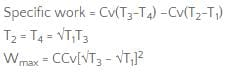QUESTION: 3

### Match List-I (Details of process of the cycle) with List-II (Name of the cycle) and select correct answer using the codes given below the lists: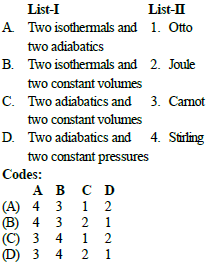Solution:
QUESTION: 4

In diesel engine the diesel fuel injected into cylinder would burn instantly at about compressed air temperature of

Solution:
QUESTION: 5

Match List-I (name of cycles) with List-II (pV- diagram) and select correct answer using the codes given below the lists: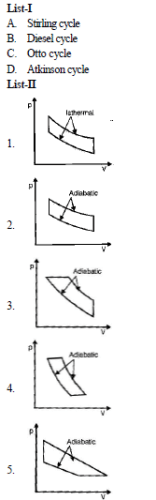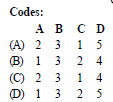Solution:
QUESTION: 6

Match List-I (Cycle operating between fixed temperature limits) with List-II (Characteristics of cycle efficiency h temperature) and select correct answer using the codes given below the lists: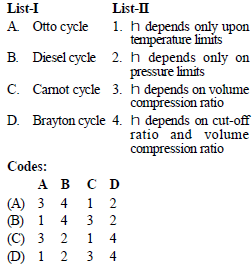Solution:
QUESTION: 7

The correct sequence of the given four cycles on T-s plane in Figure (1), (2), (3), (4) is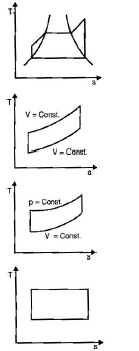Solution:
QUESTION: 8

Match List-I (cycles) with List-II (Processes) and select correct answer using the codes given below the lists: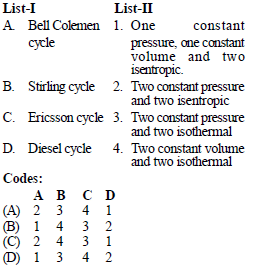Solution:
QUESTION: 9

The correct sequence of the cycles given in the above T-s diagram is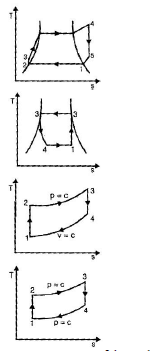Solution:
QUESTION: 10

The operation of forcing additional air under pressure in the engine cylinder is known as

Solution:
QUESTION: 11

Which cycle consists of two reversible isotherms and two reversible isobars?

Solution:
QUESTION: 12

Match List-I (Gas cycles) with List-II (Thermodynamic coordinates) and select correct answer using the codes given below the lists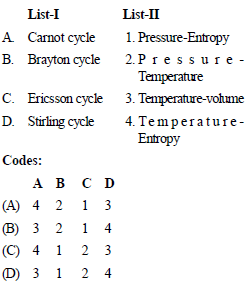Solution:
QUESTION: 13

In SI engines for higher thermal efficiency

Solution: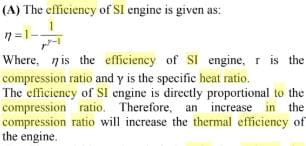QUESTION: 14

The two reference fuels used for cetane rating are

Solution:
QUESTION: 15

In some carburetors, meter rod and economiser device is used for

Solution:
QUESTION: 16

In the operation of four-stoke diesel engines, the term 'squish' refers to the

Solution:
QUESTION: 17

The stoichiometric air-fuel ratio for petrol is 15 : 1. what is the air-fuel ratio regarded for maximum power?

Solution:
QUESTION: 18

In a diesel engine, the fuel is ignited by

Solution:
QUESTION: 19

For a conventional SI engine, the value of fuelair ratio in the normal operating range is

Solution:

Explanation : The normal operating range:

For a conventional SI engine using gasoline fuel.

12 ≤ A/F ≤ 18

(0.056 ≤ F/A ≤0.083)

QUESTION: 20

The Stoichiometric air-fuel ratio by volume for combustion of carbon monoxide in air is

Solution:
QUESTION: 21

Pistons of diesel engines are usually cooled by

Solution:
QUESTION: 22

Air injected in IC engines refers to injection of

Solution:
QUESTION: 23

The object of providing masked inlet valve in the air passes of compression ignition engines is to

Solution:
QUESTION: 24

which one of the following types of swirls is generated by a precombustion chamber in the diesel engine ?

Solution:
QUESTION: 25

Match List-I (Operation mode of SI engine) with List-II (Appropriate Air-fuel Ratio) and select correct answer using the codes given below the lists: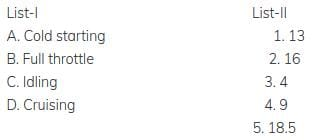Solution:Use Code STAYHOME200 and get INR 200 additional OFF Use Coupon Code